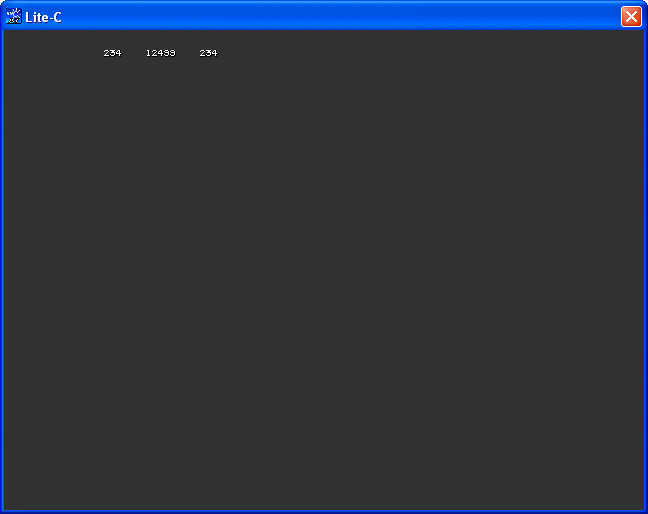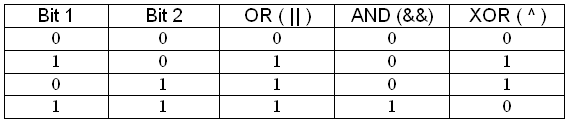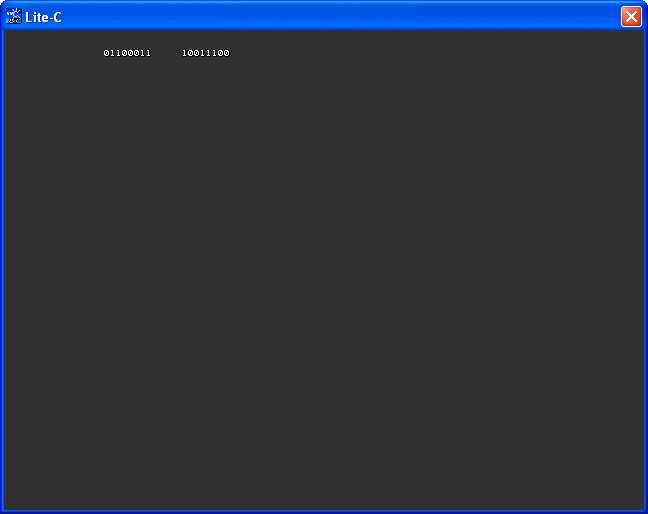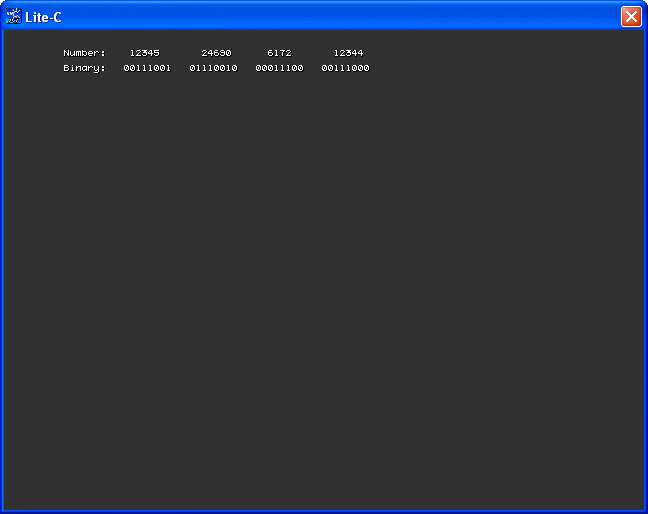lite-C 101
 This month we are going to learn about operators. You already know some of them, like && or || so we won't discuss how they work here.   Start lite-C, and then open the operators1.c file.#include  #include    STRING* value_str = "                        "; STRING* temp_str = "      ";   TEXT* values_txt = {        layer = 15;        pos_x = 100;        pos_y = 20;        string (value_str);        flags = VISIBLE; }    function main() {        video_mode = 6;        vec_set(screen_color, vector(55, 55, 55));          int i = 234;        str_cpy(value_str, str_for_num(temp_str, i));        i = i ^ 12345;        str_cat(value_str, "    ");        str_cat(value_str, str_for_num(temp_str, i));        i = i ^ 12345;        str_cat(value_str, "    ");        str_cat(value_str, str_for_num(temp_str, i)); }   You might have noticed that I keep using texts to display the numerical values on the screen; there are several reasons for that, but the most important reason is the increased  precision when compared to the "digits" instructions.   The code displays the value of a variable, then its XOR (eXclusive OR) value, and then it XORs the variable one more time. Let's discuss the following lines of code; they are the important ones:          int i = 234;        str_cpy(value_str, str_for_num(temp_str, i));        i = i ^ 12345;        str_cat(value_str, "    ");        str_cat(value_str, str_for_num(temp_str, i));        i = i ^ 12345;        str_cat(value_str, "    ");        str_cat(value_str, str_for_num(temp_str, i));   We define an integer, and then we display it on the screen by converting its numerical value to a string using str_for_num. Don't forget that values_txt is always visible,  so anything we throw at value_str will be displayed instantly.    The XOR operator can toggle the bits for any variable; since you are my friend, I have created a table that shows how the OR, AND and XOR operators work just for you.If you take a look at the XOR column, you can see that the result is set to 1 whenever one of the bits is "1" and the other bit is "0".  In our example, we are XORing 234 and 12345; these numbers can be written in binary as 11101010 and 00111001 and the result of their XOR is 11010011 = 12499.   Before you start believing that I am one of those weird looking binary guys, allow me to tell you that I have created a small function that converts the numbers to their  binary representations; you will get to see its code very soon.   One interesting property of the XOR operator is this: if we XOR a number twice, we get the initial number. This makes it very easy to encrypt some data by applying  a XOR operation to it; this way, when we want to decrypt the information, we XOR it one more time and that's it!  You can see this very clearly in our example; the number is XORed  twice and the final result is identical with the initial number.   Let's try another example; open the operators2.c file and run it.#include  #include    STRING* value_str = "                                        "; // stores up to 40 characters   TEXT* values_txt = {        layer = 15;        pos_x = 100;        pos_y = 20;        string (value_str);        flags = VISIBLE; }    function main() {        video_mode = 6;        vec_set(screen_color, vector(55, 55, 55));          int a = 99;        int i;                str_cpy(value_str, ""); // reset the string        for(i = 128; i > 0; i = i / 2)         {                if (i & a)                {                        str_cat(value_str, "1");                }                else                {                        str_cat(value_str, "0");                }        }        str_cat(value_str, "     "); // add a few spaces        a = ~a;        for(i = 128; i > 0; i = i / 2)        {                if (i & a)                {                        str_cat(value_str, "1");                }                else                {                        str_cat(value_str, "0");                }        } }   The interesting things happen inside function main; in fact, the most important line of code is this:   a = ~a;   The "~" operator inverts all the bits for our variable; if the binary value of a would be 10101010, after this operation it would be changed to 01010101, get it? The code includes two identical "for" loops which convert our number to binary before and after applying the bitwise inversion operator. Let's examine one of the loops:          for(i = 128; i > 0; i = i / 2)         {                if (i & a)                {                        str_cat(value_str, "1");                }                else                {                        str_cat(value_str, "0");                }        }   If we have a number that is written using 8 bits (a byte) the bit on the left side of the byte is 128; this will be the starting value for i in our loop. As the loop is run over and over, i is divided by 2, changing its value to 64, 32, 16... This way every time when the loop is run, we test a bit from a and see if it is set to 0 or 1. The test is done using the "&" operator, which is similar to the good old "&&" operator.   I know that I might have lost you, so here's what is happening with i and a inside our loop: at first, i = 128 and a = 99; the binary value of a is 01100011 (but we don't know that yet - that's how it is stored in our computer's memory).   The loop starts with i = 128 = 10000000, so i & a = 128 & 99 = 10000000 & 01100011 = 0. Check my table and you will see that the result of the AND operation would be 1 only if both bits from the left side of the numbers would be 1.   The loop was run once here, so the value of i is halved; i = 64 = 01000000. Now we have i & a = 64 & 99 = 01000000 & 01100011 = 1. This time, both (second) bits from the left side are 1, so the result of the & operation is 1. The following step would be i & a = 32 & 99 = 00100000 & 01100011 = 1 and so on.   The process repeats, revealing more and more bits from our number until we have got the entire byte (8 bits). Well, now that you've seen the code that does the conversion to binary, you can be a weird looking binary guy, just like me...   Time to open the operators3.c example:   #include #include   STRING* value_str = "                                                  "; // stores up to 50 characters STRING* binary_str = "                                                  "; // stores up to 50 characters STRING* temp_str = "                                                  "; // stores up to 50 characters   TEXT* values_txt = {        layer = 15;        pos_x = 60;        pos_y = 20;        string (value_str);        flags = VISIBLE; }   TEXT* binary_txt = {        layer = 15;        pos_x = 60;        pos_y = 35;        string (binary_str);        flags = VISIBLE; }   function show_binary(number) {        int i;        for(i = 128; i > 0; i = i / 2)        {                if (i & number)                {                        str_cat(binary_str, "1");                }                else                {                        str_cat(binary_str, "0");                }        } }   function main() {        video_mode = 6; // create a program window of 640x480 pixels        vec_set(screen_color, vector(55, 55, 55)); // make the background color dark        str_cpy(value_str, "Number:    ");        str_cpy(binary_str, "Binary:   ");        int my_number = 12345;        str_cat(value_str, str_for_num(temp_str, my_number));        show_binary(my_number);        str_cat(value_str, "       ");        my_number = my_number << 1;        str_cat(value_str, str_for_num(temp_str, my_number));        str_cat(binary_str, "   ");        show_binary(my_number);        str_cat(value_str, "      ");        my_number = my_number >> 2;        str_cat(value_str, str_for_num(temp_str, my_number));        str_cat(binary_str, "   ");        show_binary(my_number);        str_cat(value_str, "       ");        my_number = my_number << 1;        str_cat(value_str, str_for_num(temp_str, my_number));        str_cat(binary_str, "   ");        show_binary(my_number); }   The code appears to be bigger than usual, but most of it consists of 2 text definitions and a function named show_binary( ) which converts a number to its binary representation. We have discussed how that "for" loop works so we don't need to do it again. Let's run the code and see what happens on the screen.You can see that we have two rows: one of them displays the numbers and the other one their binary representation. We are going to learn about the bitwise shifting operators: << (bitwise left shift) and >> (bitwise right shift). All the important stuff can be found inside function main( ) so let's examine it one more time.   function main() {        video_mode = 6; // create a program window of 640x480 pixels        vec_set(screen_color, vector(55, 55, 55)); // make the background color dark        str_cpy(value_str, "Number:    ");        str_cpy(binary_str, "Binary:   ");        int my_number = 12345;        str_cat(value_str, str_for_num(temp_str, my_number));        show_binary(my_number);        str_cat(value_str, "       ");        my_number = my_number << 1;        str_cat(value_str, str_for_num(temp_str, my_number));        str_cat(binary_str, "   ");        show_binary(my_number);        str_cat(value_str, "      ");        my_number = my_number >> 2;        str_cat(value_str, str_for_num(temp_str, my_number));        str_cat(binary_str, "   ");        show_binary(my_number);        str_cat(value_str, "       ");        my_number = my_number << 1;        str_cat(value_str, str_for_num(temp_str, my_number));        str_cat(binary_str, "   ");        show_binary(my_number); }   These shifting operators work fine with integers and chars. When we shift a number to the left, all its bits will be moved towards left and a zero will be added to the right. When we shift a number to the right, all its bits will be moved towards right and a zero will be added to the left side of the number. In both cases, the bits that are moved outside the variable boundaries are lost.   Whenever we shift a variable to the right, we are in fact dividing that variable by 2; when we shift a variable to the left, we are multiplying its value by 2. These operators are widely used because they are much faster than * or /.   Our example starts with a my_number value of 12345; the binary representation of the number is 00111001. Then, we encounter the first bitwise left shift operation:          my_number = my_number << 1;   The value of the number is now double; my_number = 24690 and its binary representation is 01110010. You can see that the bits have been shifted towards left and a zero has been added to the right side of the number.          my_number = my_number >> 2;   As soon as this line is run, the number is shifted 2 bits towards the right side; its value is now 24690 / 4 = 6172. In fact, the exact result of the division would be 6172.5 but since we are using integers only 6172 is stored. The binary representation of the number is now 00011100.          my_number = my_number << 1;   This last line tries to restore the initial value of the number; we have shifted it a bit left, 2 bits right and now a bit towards left again. The result is 6172 * 2 = 12344, slightly different from the initial my_number = 12345 value. The binary representation of the number is now 00111000.   Now we know how to increase the speed of our show_binary( ) function by simply replacing "i = i / 2" with "i = i >> 1".   function show_binary(number) // this function will run faster than the one from my example {        int i;        for(i = 128; i > 0; i = i >> 1)        {                if (i & number)                {                        str_cat(binary_str, "1");                }                else                {                        str_cat(binary_str, "0");                }        } }   That's pretty much it for today; we have learned quite a few new things so you can enjoy your favorite binary soda...